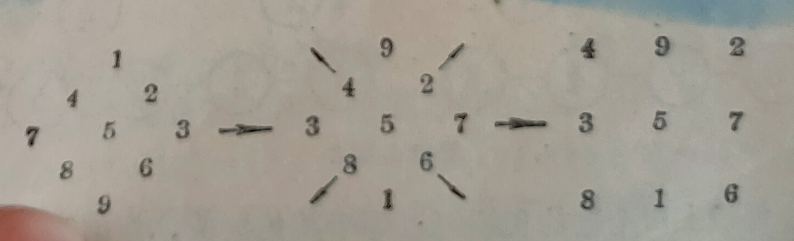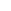# 有益的游戏-填数游戏 九宫图## 答案及解题思路

 X11 X12 X13 X21 X22 X23 X31 X32 X33

(x11 +x12+x13)+(x21+x22+x23)+(x31 +x32 +x33)
+(x13+x22+x31)+(x11+x22+x33)=75

(x11+x12+x13)+(x31+x32+x33)+(x11+x22+x33)
+（x13+x23+x33）=60

1+6+8   2+6+7
2+4+9   3+4+8

 2 7 6 9 5 1 4 3 8https://baike.baidu.com/item/%E4%B9%9D%E5%AE%AB%E5%9B%BE%E5%88%86%E6%9E%90%E6%B3%95/4165444

### 目录[+]